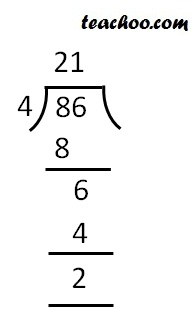Divisibility Tests - Divisibility by 4, 8

Chapter 3 Class 6 Playing with Numbers
Concept wise

A number is divisible by 4

If its last two digits are divisible by 4

## Example

#### 212

Last two digits = 12

Since 12 is divisible 4,

212 is divisible by 4

#### 1936

Last two digits = 36

Since 36 is divisible by 4

1936 is divisible by 4

#### 4612

Last two digits = 12

Since 12 is divisible by 4

4612 is divisible by 4

#### 286

Last two digits = 86

Since 86 is not divisible by 4

286 is not divisible by 4#### 9532

Last two Digits = 32

Since 32 is divisible by 4

9532 is divisible by 4

#### 800

Last Two Digits = 00

= 0

And 0 is divisible by any number

∴ 0 is divisible by 4 (as 0/4 = 0, reminder is 0)

Thus 800 is divisible by 4

Learn in your speed, with individual attention - Teachoo Maths 1-on-1 Class